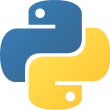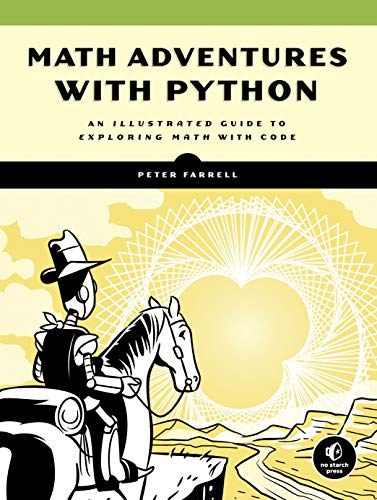Language
• Python 3Reading time
• Approximately 30 daysWhat you will learn
• Programming Basics and Python Syntax
• Numerical Programming and Data MiningAuthor
• Peter FarrellPublished
• 4 years, 2 months agoPackages you will be introduced to
• turtleLearn math by getting creative with code! Use the Python programming language to transform learning high school-level math topics like algebra, geometry, trigonometry, and calculus!

Math Adventures with Python will show you how to harness the power of programming to keep math relevant and fun. With the aid of the Python programming language, you'll learn how to visualize solutions to a range of math problems as you use code to explore key mathematical concepts like algebra, trigonometry, matrices, and cellular automata.

Once you've learned the programming basics like loops and variables, you'll write your own programs to solve equations quickly, make cool things like an interactive rainbow grid, and automate tedious tasks like factoring numbers and finding square roots. You'll learn how to write functions to draw and manipulate shapes, create oscillating sine waves, and solve equations graphically.

You'll also learn how to:
- Draw and transform 2D and 3D graphics with matrices
- Make colorful designs like the Mandelbrot and Julia sets with complex numbers
- Use recursion to create fractals like the Koch snowflake and the Sierpinski triangle
- Generate virtual sheep that graze on grass and multiply autonomously
- Crack secret codes using genetic algorithms

As you work through the book's numerous examples and increasingly challenging exercises, you'll code your own solutions, create beautiful visualizations, and see just how much more fun math can be!